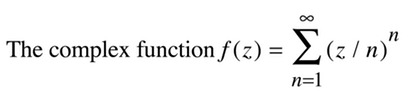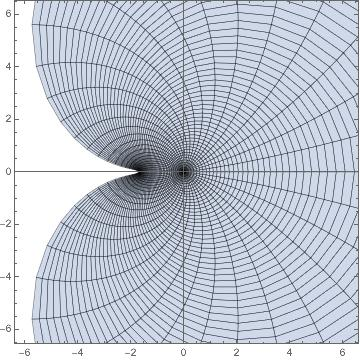I find this function interesting. The power series coefficients, (1/n^n), decrease quite rapidly, faster than 1/n!. By the root test, for example, the infinite series converges everywhere (infinite radius of convergence) so the function is an entire function (holomorphic in the entire complex plane).
To the best of my knowledge it is unproven whether the value  f(1)=1.291285997...is transcendental or even irrational. This value also arises as the integral from 0 to 1 of 1/(x^
x). See: Weisstein, Eric W. "Sophomore's Dream." From MathWorld--A Wolfram Web Resource. Sophomore's Dream.
The first plot is a parametric plot of the real and imaginary parts of f(z), (Re(f(z)),Im(f(z))), for Abs(z) between 0 and 5 and Arg(z) from 0 to 2
π. It can be hard to see a pattern here, so another approach is to decompose it into separate plots.
The second plot shows how f(z) maps the imaginary axis (i.e. points of the form 0+yi). It maps the imaginary axis into an expanding spiral shape. Increasing positive values of y map to a counterclockwise spiral shape, and negative values a clockwise one. They meet at (0,0).
The third plot shows that f(z) maps circles of small radius into oval shapes, where the positive real side expands and the negative real side is pinched in.
It can be shown that
$f(z)= ∫ 0 1 z t -tz dt MathType@MTEF@5@5@+=faaagCart1ev2aqaKnaaaaWenf2ys9wBH5garuavP1wzZbqedmvETj2BSbqefm0B1jxALjharqqtubsr4rNCHbGeaGqiVu0Je9sqqrpepC0xbbL8FesqqrFfpeea0xe9Lq=Jc9vqaqpepm0xbba9pwe9Q8fs0=yqaqpepae9pg0FirpepeKkFr0xfr=xfr=xb9Gqpi0dc9adbaqaaeGaciGaaiaabeqaamaabaabaaGcbaGaamOzaiaacIcacaWG6bGaaiykaiabg2da9maapedabaGaamOEaiaadshadaahaaWcbeGcbaGaaiylaiaadshacaWG6baaaaWcbaGaaGimaaqaaiaaigdaa0Gaey4kIipakiaadsgacaWG0baaaa@3CC5@$